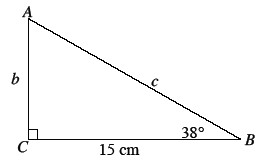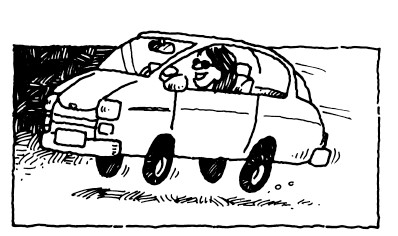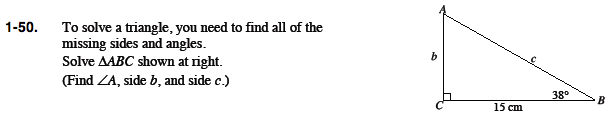### Home > PC > Chapter 1 > Lesson 1.1.4 > Problem1-50

1-50.
1. To solve a triangle, you need to find all of the missing sides and angles. Homework Help ✎
Solve ΔABC shown below
(Find ∠A, side b, and side c.)

2.Label all of the sides and angles on the triangle. Always label the sides with the lower case letter of the angle opposite it.

To make this easier, first make a table of sides and angles and fill in the information given.

You have two given angles. How do you get the third angle?

Now that you have all three angles and a side, would you use the Law of Sines or the Law of Cosines to solve for the other two sides?

Side Angle

a = 15 A =
b = B = 38°
c = C = 90°

Law of Sines

$\frac{a}{\text{sin}(A)} = \frac{b}{\text{sin}(B)} = \frac{c}{\text{sin}(C)}$

Show all work and solve for the other sides.